2.13 Variation :

Observe the below mentioned examples which we encounter in our daily life:

Example 1: If 180 men in 6 days, working 10 hours daily, can dig a trench of 60m long, 1 m wide and 1 m deep, how many days are needed for 100 men, working 8 hours a day, to dig a trench of 100m long, 1.5m wide and 1.2m deep?

Example 2: The weight of a body varies inversely as the square of its distance from the centre of the earth. If the radius of the earth is about 6380 KM, how much would a 80 KG man weigh 1600 KM above the surface of the earth?

How  can we find solutions to such problems?

Variation means change. We say things have changed with time and we hear quite often that this was not the case with our time and things have changed. We often hear that in our time the rice is used to cost few Anas  per KG, where is it  now  costs in multiples of 10 Rs. Has the price of rice increased directly with passage of time?. No. it has come down also. So raise in the price of rice or rise in the price of gold, petrol or any item has not always increased with passage of time. So price raise is not directly proportional to time. Does  the height  of a person with increase in age?  Though  height grows  continuously in the initial stage, after certain age it stops. So height does  is not directly vary with age.

2.13.1 Direct variation(Proportion)

Is there  some thing which always increase with time? Yes, distance covered by moving train or bus always increases with the time as per its speed. We also know  that

Distance travelled = Speed*time or  d=st. If time travelled is less, then distance covered is less. In this case we say distance is directly proportional to time and we write d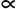t and read this relation as: d is directly proportional to t

Note that  d/t = k- a constant (speed).  Here  k is a constant which  does not change and is called 'constant of proportionality' where as d and t are variables.  Interest paid by a bank to a depositor or interest charged on a loan is directly proportional to the amount when term and rate of interest is fixed.

We know circumference  of a circle = 2pr. As the radius  of a circle increases or decreases, circumference increases or decreases. Also  note that Cr because C/r = 2p which  is a constant. Similarly Area of a circle = pr2. Thus Ar and A/r2= p. Here also area of a circle increases or decreases with increase or decrease in the radius. To know the distance between 2 places, we use maps. The distance between two places in a map is multiplied scale mentioned in the map to get actual distances(Ex 1 cm = 10 Kms). Is weight directly  proportional to age? –NO.

2.13 Problem 1 : The distance through which a body falls from rest varies as square of time it takes to fall that distance. It is known that body falls 64 cm in 2 seconds. How far does that body fall in 6 seconds?

Solution:

Note that we can not solve as in unitary method like

2sec >>> 64cm6sec  >>> (64/2)*6= 192

This is not the solution.

It is given that d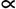t2d/t2= kk = 64/4= 16

Now k = 16= d/62=d/36d= 16*36= 576

Thus the body falls  in 576 cms in 6 seconds

2.13.2 Inverse variation(Proportion)

Have you heard about growers throwing tomatoes on the road when there is bumper crop? Why it is so? Note that:

·         When there is too much of supply of vegetables or paddy, wheat etc, the price of them crashes.

·         When there is too much of supply of fuel in the international market the price of Petrol  and Diesel drops

Examples:

1.      When more number of people are involved in a manual work, time taken to complete the job decrease

2.      We reach a state of weightlessness when we move far away from earth

In direct variation, when a  value of a variable  increases, the value of another dependant variable also increases.

However, in the examples listed above when a  value of a variable  increases, the value of another dependant variable  decreases. In such cases, product dependent on dependent variables is constant.

In other words, If  x and y are 2 variables then x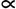1/y. We also say x  inversely varies with y and accordingly xy=k a constant.

Like this we can also have x1/y2 ,  x1/y4 , x1/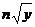. . . . and then xy2, xy4, xwill be constant respectively.

2.13 Problem 2 : When a ball is thrown upwards, the time, T seconds during which the ball remains in the air is directly proportional to the square root of the height, h meters. reached. We know T=4.47sec when h=25m.

(i)                 Find the formula for T in terms of h

(ii)               Find T when h=50

(iii)              If the ball is thrown upwards and remains in the air for 5 seconds, find the height reached.

Solution:

T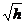T= k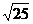4.47= 5kk = 0.894T = 0.894When h =50

T= 0.894*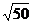= 0.894*7.07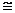6.32

When T =5= T/k = 5/0.8945.6031.36m

2.13.3 Joint Variation

Can a variation depend upon multiple variables? We know the formula for interest calculation  which for simple interest and compound interest is

SI = PTR/100

and

CI = P(1+R/100)T-P

What do we observe? we observe that Simple Interest is directly proportional to Principal P, term T and rate of interest R and Compound interest is dependent on those three variables.

We also know that  weight of a person depends upon the distance he is  away from the centre of earth.

2.13.4 Work, People, Days, hours

We know the  work done is directly proportional to number of men(M), number of days(D) and number of hours(H).

In other words,

WM, WD, WHWM*D*H or M*D*H/W = constant

2.13 Problem 3 : If 36 men can build a wall of 140M long in 21 days, how many men are required to build a similar wall of length 50M in 18 days?

Solution:

Here W1= 140, M1=36, D1=21 and given W2= 50, D2=18 'H' is number of hours though not given is equal.  we need to find M2 such that

M*D*H/W = constant

Hence 36*21*H/140 = M2*18*H/50

On solving we get M2= 15

2.13 Problem 4 : Tap A Can fill a cistern in 8 hours and tap B can empty it in 12  hours. How long will it take to fill the cistern if both of them are opened together

Solution:

Part filled in 1 hour = (1/8-1/12)= (3-2)/24= 1/24.

Time taken to fill the tank= 24 hours

2.13 Problem 5 : If 180 men in 6 days, working 10 hours daily, can dig a trench of 60m long, 1 m wide and 1 m deep, how many days are needed for 100 men, working 8 hours a day, to dig a trench of 100m long, 1.5m wide and 1.2m deep?

Solution:

Here W1= 60*1*1, M1=180, D1=6 and H1 =10, and given W2= 100*1.5*1.2, M2=100, and H2 =8,  we need to find D2 such that

M*D*H/W = constant

M1*D1*H1/W1 = M2*D2*H2/W2

Hence 180*6*10/60 = 100*D2*8*/(100*1.5*1.2)

On solving we get D2 = 40.5

2.13 Problem 6 : The weight of a body varies inversely as the square of its distance from the centre of the earth. If the radius of the earth is about 6380 KM, how much would a 80 KG man weigh 1600 KM above the surface of the earth?

Solution:

Here W1/d2

Weight of the person when he is on earth  = 80 KG. d1 =Radius  of earth=6380 KM

Let W2  be his weight when he is 1600 KM above the earth's surfaceW1 d12= W2 d22

80*63802= W2*79802

On solving we get  W2= 51.14

2.13 Problem 7 : Suppose A alone can perform a work in 5 days more than A and B working together. Suppose further, B working alone can complete the same work in 20 days more than A and B working together. Find out how much time it will take A and B both working together?

Solution:

Let x be the time taken by  A and B both working together to perform the work.

It is given that A alone can complete the work in x+5 days

It is also given that B alone can complete the work in x+20 days

From formula, The time taken by both A and B working together is(=x)= (x+5)*(x+20)/{(x+5)+(x+20)}x= x2+25x+100/2x+252x2+25x= x2+25x+100x2=100x=10

The time taken by both A and B,  when they work together to complete the work is 10 days

Verification:

Time taken by A alone to complete the work = x+5 = 15 days

Time taken by B alone  to complete the work = x+20 = 30 days

From formula, the time taken by both A and B, when they work together= 30*15/45= 10

2.13 Problem 8 : It takes 1 hour to fill a tank by a pump A. 1 hour and 40 minutes using a pump B. A third pump C takes average time of pump A and B to fill up the tank. Suppose the pumps A and B are started together and 2 pumps of capacity C are used to drain  the water. Will the tank ever get filled? If so in how much time it gets filled ?

Solution:

Time taken by pump A = 60 Minutes

Time taken by pump B = 100 Minutes

Time taken by pump C = 80 Minutes

Let t be the time  taken to fill up the tank

By formula

1/t = 1/60+1/100- 2(1/80)

= 1/60+1/100-1/40= (10+6-15)/600 = 1/600

Time taken to fill up the tank = 600 minutes= 10 hours

Summary of learning

xy , x/y = k a constant

x1/y. xy=k a constant

WM*D*H or M*D*H/W = k a constant